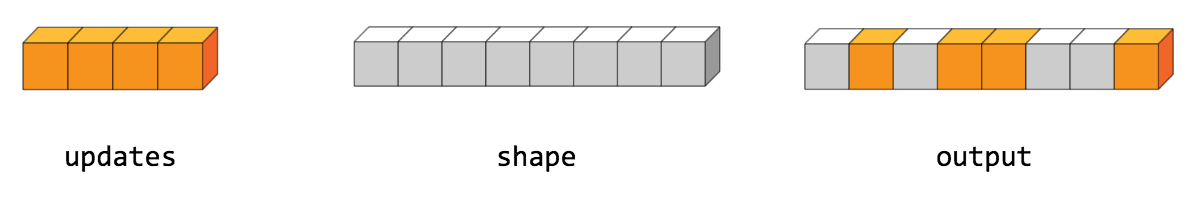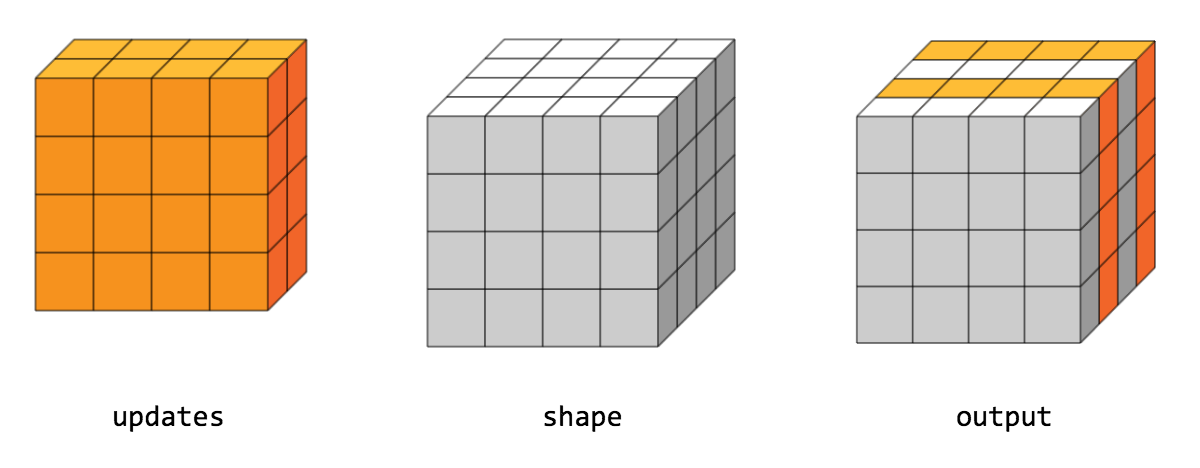# ScatterNd

public final class ScatterNd

Scatters `updates` into a tensor of shape `shape` according to `indices`.

Scatter sparse `updates` according to individual values at the specified `indices`. This op returns an output tensor with the `shape` you specify. This op is the inverse of the `tf.gather_nd` operator which extracts values or slices from a given tensor.

This operation is similar to `tf.tensor_scatter_nd_add`, except that the tensor is zero-initialized. Calling `tf.scatter_nd(indices, updates, shape)` is identical to calling `tf.tensor_scatter_nd_add(tf.zeros(shape, updates.dtype), indices, updates)`

If `indices` contains duplicates, the associated `updates` are accumulated (summed) into the output tensor.

WARNING: For floating-point data types, the output may be nondeterministic. This is because the order in which the updates are applied is nondeterministic and when floating-point numbers are added in different orders the resulting numerical approximation error can be slightly different. However, the output will be deterministic if op determinism is enabled via `tf.config.experimental.enable_op_determinism`.

`indices` is an integer tensor containing indices into the output tensor. The last dimension of `indices` can be at most the rank of `shape`:

indices.shape[-1] <= shape.rank

The last dimension of `indices` corresponds to indices of elements (if `indices.shape[-1] = shape.rank`) or slices (if `indices.shape[-1] < shape.rank`) along dimension `indices.shape[-1]` of `shape`.

`updates` is a tensor with shape:

indices.shape[:-1] + shape[indices.shape[-1]:]

The simplest form of the scatter op is to insert individual elements in a tensor by index. Consider an example where you want to insert 4 scattered elements in a rank-1 tensor with 8 elements.In Python, this scatter operation would look like this:

``````indices = tf.constant([, , , ])
updates = tf.constant([9, 10, 11, 12])
shape = tf.constant()
print(scatter)
``````
The resulting tensor would look like this:

[0, 11, 0, 10, 9, 0, 0, 12]

You can also insert entire slices of a higher rank tensor all at once. For example, you can insert two slices in the first dimension of a rank-3 tensor with two matrices of new values.In Python, this scatter operation would look like this:

``````indices = tf.constant([, ])
updates = tf.constant([[[5, 5, 5, 5], [6, 6, 6, 6],
[7, 7, 7, 7], [8, 8, 8, 8]],
[[5, 5, 5, 5], [6, 6, 6, 6],
[7, 7, 7, 7], [8, 8, 8, 8]]])
shape = tf.constant([4, 4, 4])
print(scatter)
``````
The resulting tensor would look like this:

[[[0, 0, 0, 0], [0, 0, 0, 0], [0, 0, 0, 0], [0, 0, 0, 0]], [[5, 5, 5, 5], [6, 6, 6, 6], [7, 7, 7, 7], [8, 8, 8, 8]], [[0, 0, 0, 0], [0, 0, 0, 0], [0, 0, 0, 0], [0, 0, 0, 0]], [[5, 5, 5, 5], [6, 6, 6, 6], [7, 7, 7, 7], [8, 8, 8, 8]]]

Note that on CPU, if an out of bound index is found, an error is returned. On GPU, if an out of bound index is found, the index is ignored.

### Public Methods

 Output asOutput() Returns the symbolic handle of a tensor. static ScatterNd create(Scope scope, Operand indices, Operand updates, Operand shape) Factory method to create a class wrapping a new ScatterNd operation. Output output() A new tensor with the given shape and updates applied according to the indices.

## Public Methods

#### public Output<U> asOutput()

Returns the symbolic handle of a tensor.

Inputs to TensorFlow operations are outputs of another TensorFlow operation. This method is used to obtain a symbolic handle that represents the computation of the input.

#### public static ScatterNd<U> create(Scope scope, Operand<T> indices, Operand<U> updates, Operand<T> shape)

Factory method to create a class wrapping a new ScatterNd operation.

##### Parameters
scope current scope Tensor of indices. Values to scatter into the output tensor. 1-D. The shape of the output tensor.
##### Returns
• a new instance of ScatterNd

#### public Output<U> output()

A new tensor with the given shape and updates applied according to the indices.

[{ "type": "thumb-down", "id": "missingTheInformationINeed", "label":"Missing the information I need" },{ "type": "thumb-down", "id": "tooComplicatedTooManySteps", "label":"Too complicated / too many steps" },{ "type": "thumb-down", "id": "outOfDate", "label":"Out of date" },{ "type": "thumb-down", "id": "samplesCodeIssue", "label":"Samples / code issue" },{ "type": "thumb-down", "id": "otherDown", "label":"Other" }]
[{ "type": "thumb-up", "id": "easyToUnderstand", "label":"Easy to understand" },{ "type": "thumb-up", "id": "solvedMyProblem", "label":"Solved my problem" },{ "type": "thumb-up", "id": "otherUp", "label":"Other" }]